# R S Aggarwal Solutions for Class 11 Maths Chapter 25 Applications of Conic Sections

R S Aggarwal Class 11 Solutions for the Chapter 25 Applications of Conic Sections are given here in detail. RS Aggarwal Solutions are solved by experts to build the basics and in-depth understanding of the fundamental Maths concepts among the students. In this chapter, students will learn how to solve real life problems using conic sections. In order to strengthen your basic fundamentals on various Maths concepts, students must practice R S Aggarwal class 11 Solutions. Click on the link below to download solutions for chapter 25 exercise questions.

## Download PDF of R S Aggarwal Solutions for Class 11 Maths Chapter 25 Applications of Conic Sections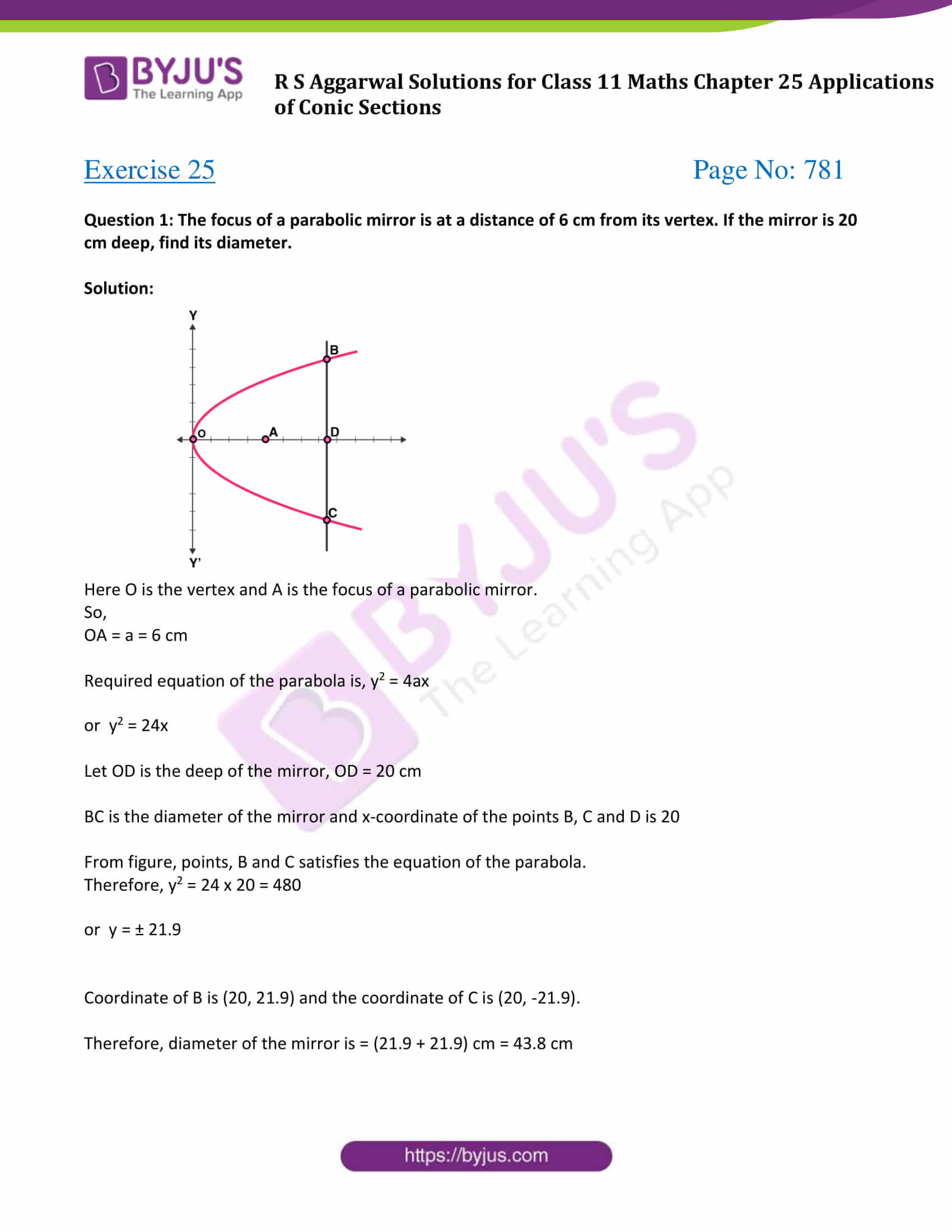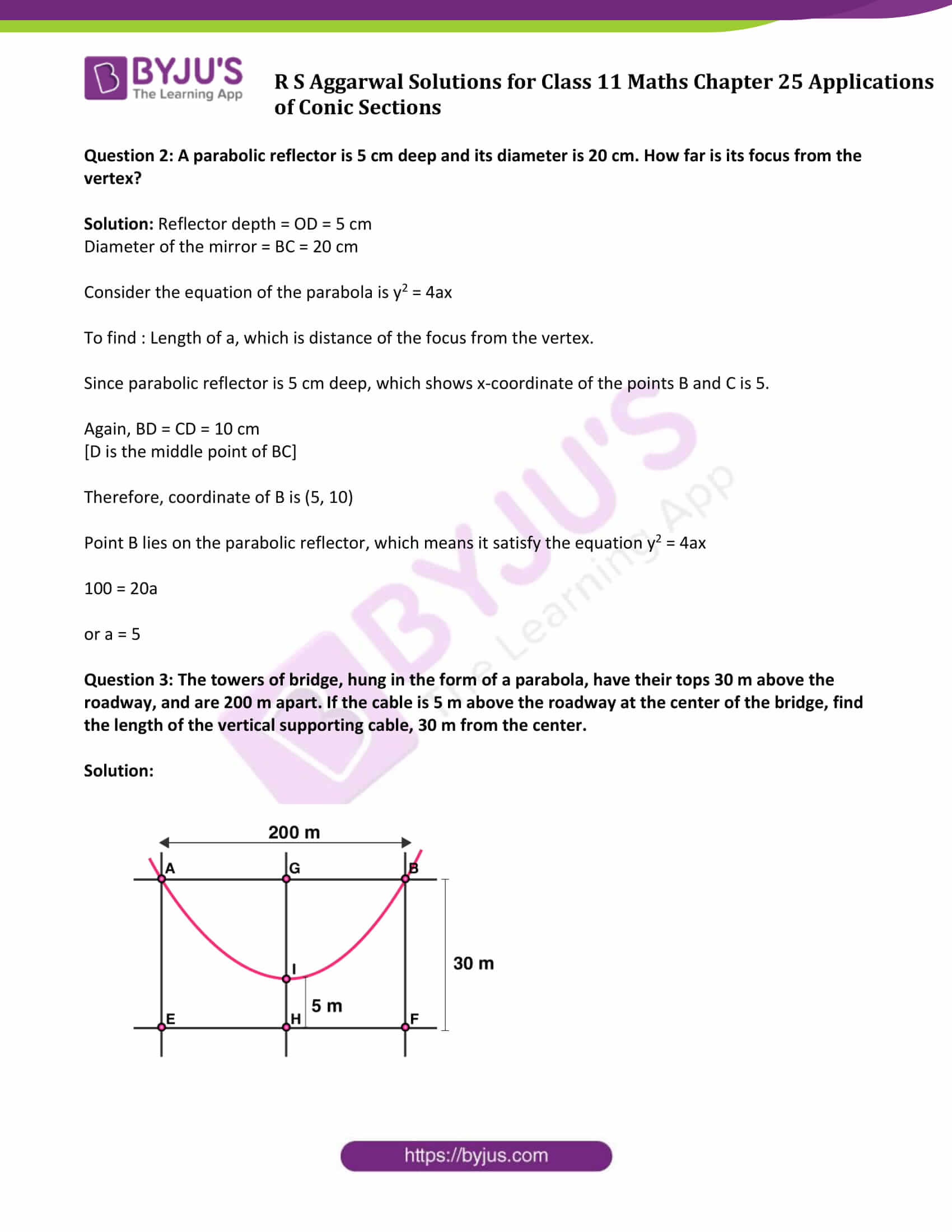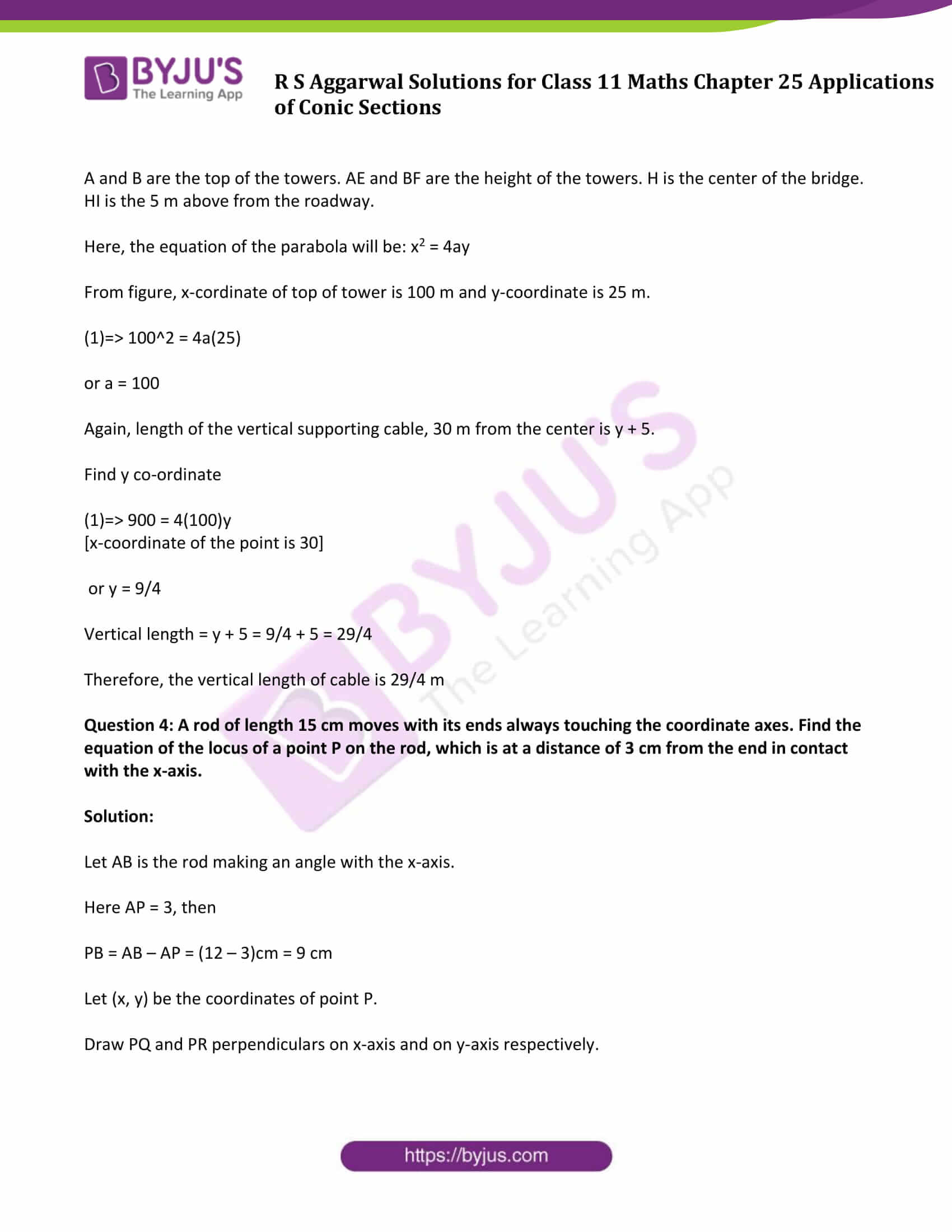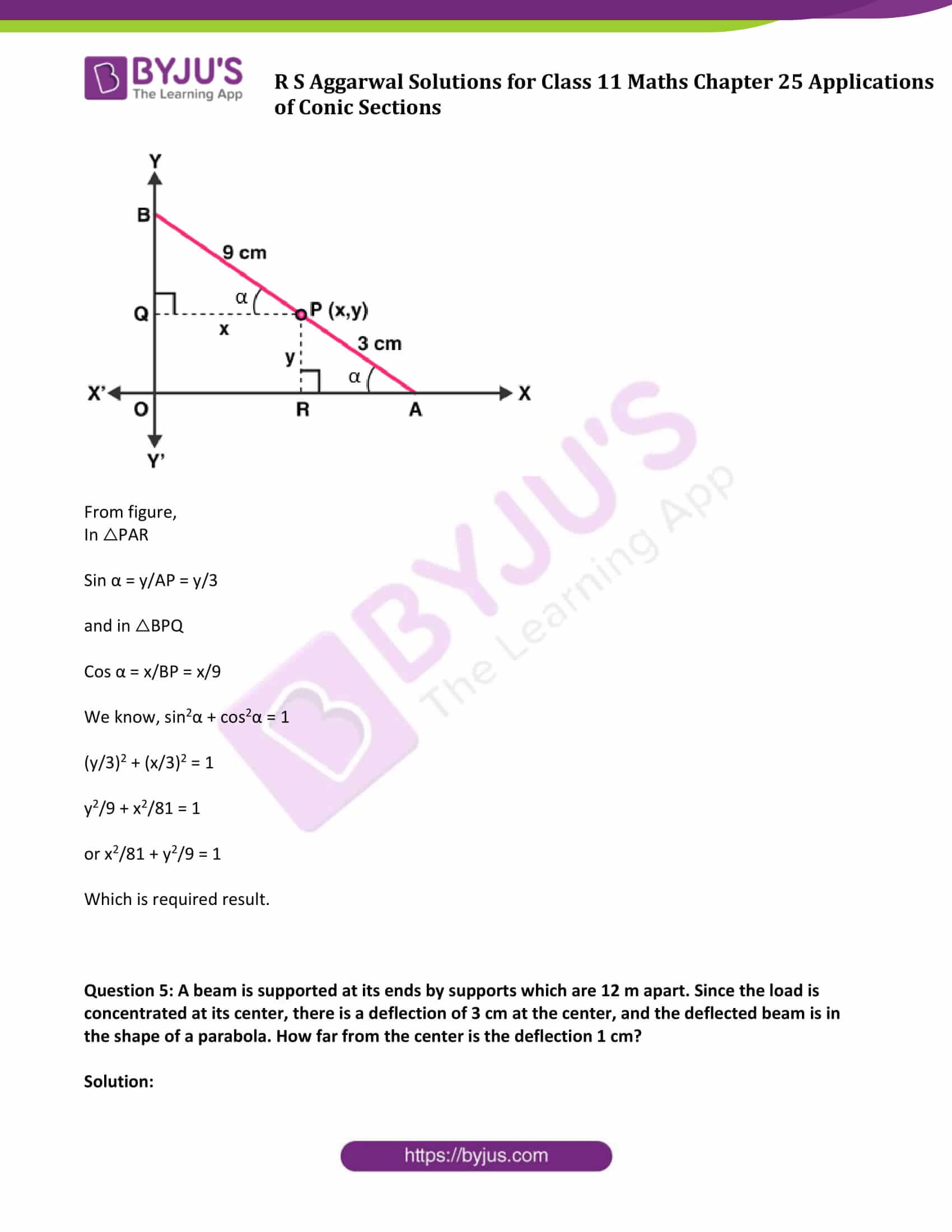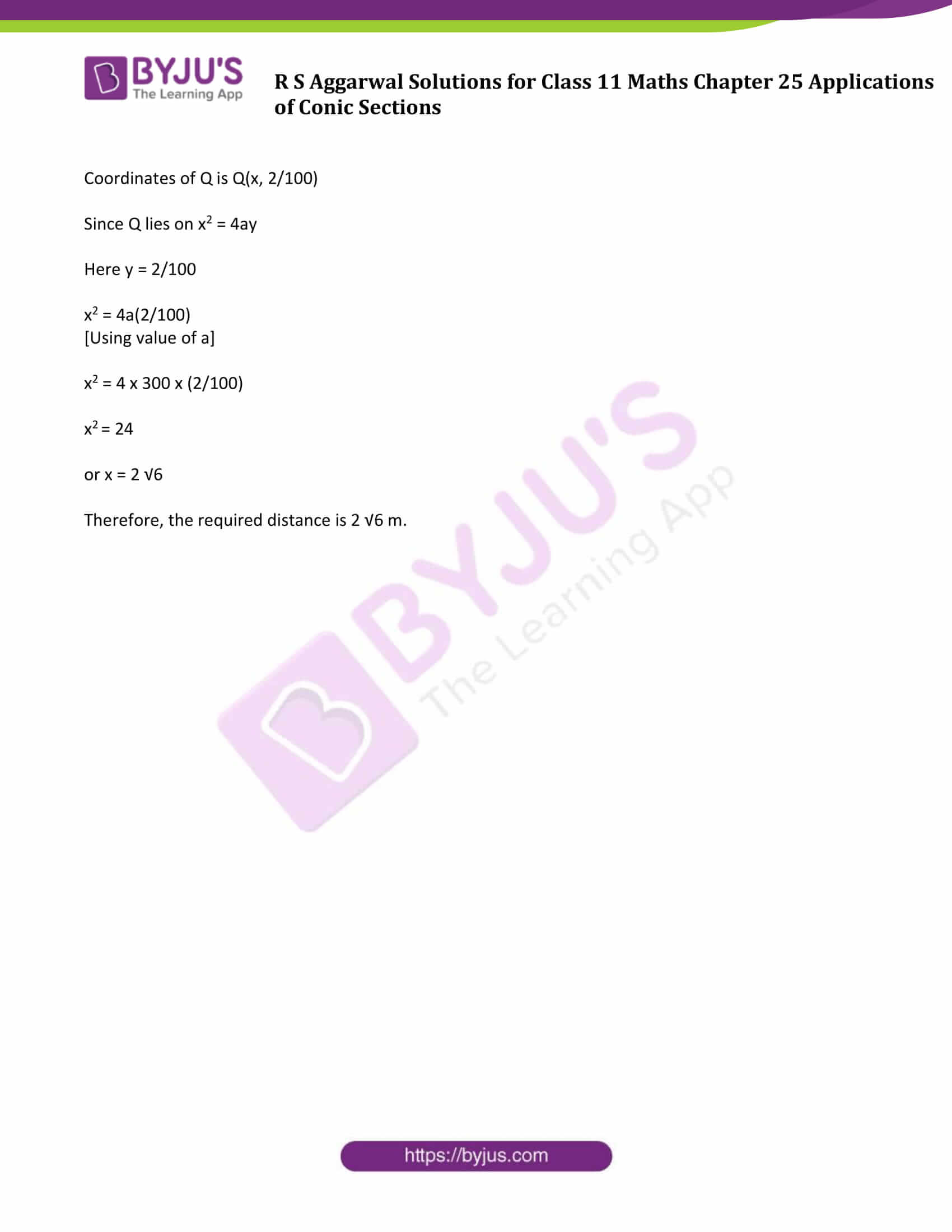### Access Answers of R S Aggarwal Solutions for Class 11 Maths Chapter 25 Applications of Conic Sections

Exercise 25 Page No: 781

Question 1: The focus of a parabolic mirror is at a distance of 6 cm from its vertex. If the mirror is 20 cm deep, find its diameter.

Solution: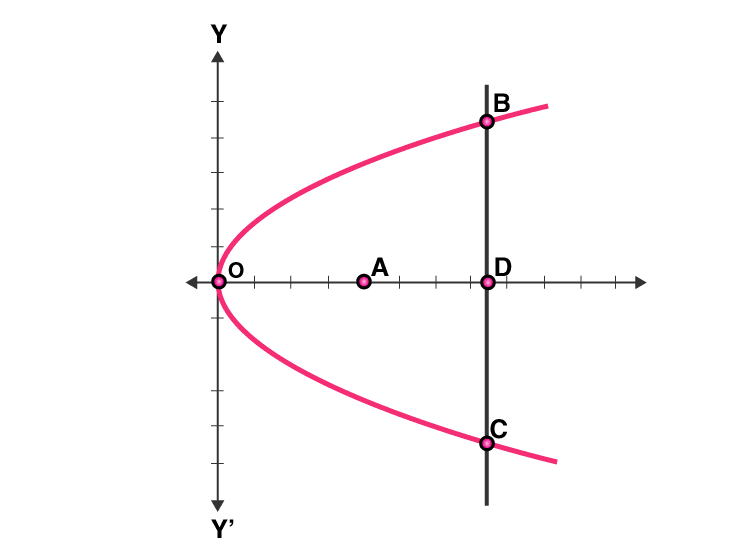Here O is the vertex and A is the focus of a parabolic mirror.

So,

OA = a = 6 cm

Required equation of the parabola is, y2 = 4ax

or y2 = 24x

Let OD is the deep of the mirror, OD = 20 cm

BC is the diameter of the mirror and x-coordinate of the points B, C and D is 20

From figure, points, B and C satisfies the equation of the parabola.

Therefore, y2 = 24 x 20 = 480

or y = ± 21.9

Coordinate of B is (20, 21.9) and the coordinate of C is (20, -21.9).

Therefore, diameter of the mirror is = (21.9 + 21.9) cm = 43.8 cm

Question 2: A parabolic reflector is 5 cm deep and its diameter is 20 cm. How far is its focus from the vertex?

Solution: Reflector depth = OD = 5 cm

Diameter of the mirror = BC = 20 cm

Consider the equation of the parabola is y2 = 4ax

To find : Length of a, which is distance of the focus from the vertex.

Since parabolic reflector is 5 cm deep, which shows x-coordinate of the points B and C is 5.

Again, BD = CD = 10 cm

[D is the middle point of BC]

Therefore, coordinate of B is (5, 10)

Point B lies on the parabolic reflector, which means it satisfy the equation y2 = 4ax

100 = 20a

or a = 5

Question 3: The towers of bridge, hung in the form of a parabola, have their tops 30 m above the roadway, and are 200 m apart. If the cable is 5 m above the roadway at the center of the bridge, find the length of the vertical supporting cable, 30 m from the center.

Solution: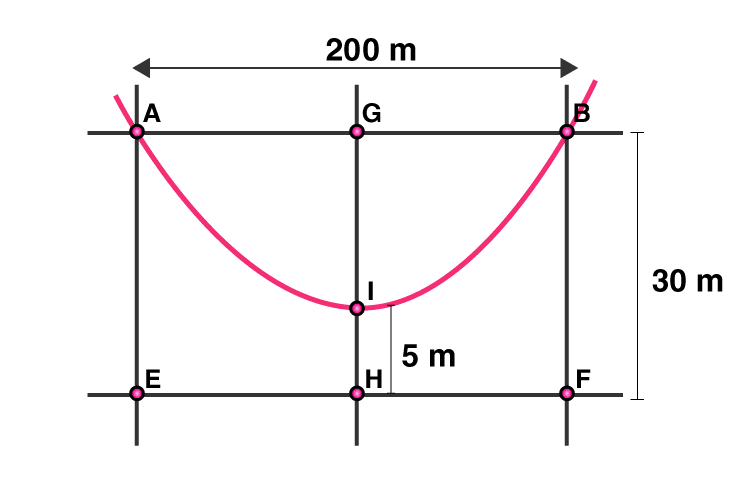A and B are the top of the towers. AE and BF are the height of the towers. H is the center of the bridge. HI is the 5 m above from the roadway.

Here, the equation of the parabola will be: x2 = 4ay

From figure, x-cordinate of top of tower is 100 m and y-coordinate is 25 m.

(1)=> 100^2 = 4a(25)

or a = 100

Again, length of the vertical supporting cable, 30 m from the center is y + 5.

Find y co-ordinate

(1)=> 900 = 4(100)y

[x-coordinate of the point is 30]

or y = 9/4

Vertical length = y + 5 = 9/4 + 5 = 29/4

Therefore, the vertical length of cable is 29/4 m

Question 4: A rod of length 15 cm moves with its ends always touching the coordinate axes. Find the equation of the locus of a point P on the rod, which is at a distance of 3 cm from the end in contact with the x-axis.

Solution:

Let AB is the rod making an angle with the x-axis.

Here AP = 3, then

PB = AB – AP = (12 – 3)cm = 9 cm

Let (x, y) be the coordinates of point P.

Draw PQ and PR perpendiculars on x-axis and on y-axis respectively.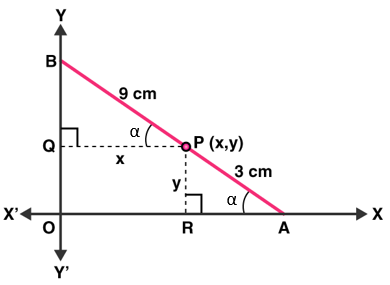From figure,

In △PAR

Sin α = y/AP = y/3

and in △BPQ

Cos α = x/BP = x/9

We know, sin2α + cos2α = 1

(y/3)2 + (x/3)2 = 1

y2/9 + x2/81 = 1

or x2/81 + y2/9 = 1

Which is required result.

Question 5: A beam is supported at its ends by supports which are 12 m apart. Since the load is concentrated at its center, there is a deflection of 3 cm at the center, and the deflected beam is in the shape of a parabola. How far from the center is the deflection 1 cm?

Solution: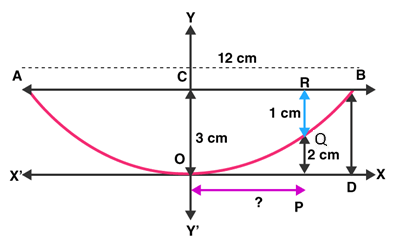Beam is always facing upwards with the axis vertical.

Here, the equation of the parabola is: x2 = 4ay …(1)

Find the coordinate of B:

Width of beam = AB = 12 m

BC = 12/2 = 6 m

[Parabola is symmetric about its axis]

Again, there is deflection of 3 cm at the center. (given)

BD = OC = 3 cm or 3/100 m

Point B is B(6, 3/100)

Since B lies on x2 = 4ay

Here x = 6 and y = 3/100

36 = 12/100 a

or a = 300 m

Now, find how far from the center is the deflection 1 cm

From figure, RQ = 1 cm

QP = (3-1)cm = 2cm or 2/100 m

Let OP = x

Coordinates of Q is Q(x, 2/100)

Since Q lies on x2 = 4ay

Here y = 2/100

x2 = 4a(2/100)

[Using value of a]

x2 = 4 x 300 x (2/100)

x2 = 24

or x = 2 √6

Therefore, the required distance is 2 √6 m.

## R S Aggarwal Solutions for Chapter 25 Applications of Conic Sections

R S Aggarwal Chapter 25 – Applications of Conic Sections, contains the answers to all the questions listed in this chapter. All exercise questions are prepared by subject experts to help students in their endeavour to develop a better understanding and score well in examinations. For more details, students can practice R S Aggarwal Class 11 Chapter 25 solutions.